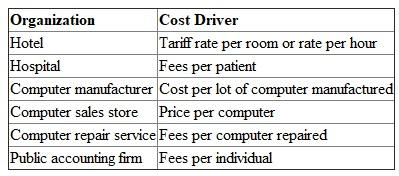# Quiz 6: Activity Analysis, Cost Behavior, and Cost Estimation

Business

Cost Behavior The cost behavior is the examining of the behavior of cost whether the cost varies with variation in production or remains constant. In other words, the cost behavior is measured in terms of what is the nature of the cost. It may be the fixed cost, variable cost, or semi variable cost. The cost estimation helps in the analysis of the cost behavior that further helps in the cost prediction for cost unit for given specification. Planning made keeping in mind the information generated by analysis of the cost behavior would be more accurate. Budgets and estimates prepared on the basis of this information would be more reliable. Control over various activities and cost to be incurred is efficient by keeping in mind the nature of cost. More planning and controlling activities are required where majority of the costs are allocated. Further, distinction made between the fixed and variable cost would be important in various decisions making. Decisions such as buy or produce, continuity of product, shut down decision, profitability of product, break-even analysis etc. are based on the cost classified by analysis of the cost behavior. Thus, the cost behavior is important in planning, controlling, and decision making.

(a) Cost Estimation Cost estimation is the process of estimating cost of the unit produced or service provided on the basis of standards set for the production activity or service activity. Cost estimation helps in the analysis of the cost behavior. (b) Cost Behavior The cost behavior is the examining of the behavior of cost whether the cost varies with variation in production or remains constant. In other words, the cost behavior is measured in terms of what is the nature of cost. It may be the fixed cost, variable cost, or semi variable cost. (c) Cost Prediction Cost prediction is a process to determine costs or computing costs on the basis of specification of the cost unit to be produced or service unit to be provided in advance before actual production or service starts. Relationship Cost estimation helps in the analysis of the cost behavior that further helps in the cost prediction for cost unit for given specification.

The following cost driver for the cost unit may be used for respective industries.